American Journal of Modern Physics
Volume 4, Issue 5, September 2015, Pages: 221-231

Quantum Mechanics in Space and Time

Fosco Ruzzene

Department of Econometrics and Business Statistics, Monash University (Retired), Caulfield East, Australia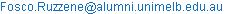Fosco Ruzzene. Quantum Mechanics in Space and Time. American Journal of Modern Physics. Vol. 4, No. 5, 2015, pp. 221-231. doi: 10.11648/j.ajmp.20150405.12

Abstract: The possibility that quantum mechanics is foundationally the same as classical theories in explaining phenomena in space and time is postulated. Such a view is motivated by interpreting the experimental violation of Bell inequalities as resulting from questions of geometry and algebraic representation of variables, and thereby the structure of space, rather than realism or locality. While time remains Euclidean in the proposed new structure, space is described by Projective geometry. A dual geometry facilitates description of a physically real quantum particle trajectory. Implications for the physical basis of Bohmian mechanics is briefly examined, and found that the hidden variables pilot-wave model is local. Conceptually, the consequence of this proposal is that quantum mechanics has common ground with relativity as ultimately geometrical. This permits the derivation of physically meaningful quantum Lorentz transformations. Departure from classical notions of measurability is discussed.

Keywords: Bell Inequalities, Non-Metric Space, Projective Geometry, Bohmian Mechanics, Quantum Lorentz Transformations, Measurability

Contents

1. Introduction

Bell’s theory is considered central to questions on the foundations of quantum mechanics . Motivated by the EPR paradox, Bell constructed experimentally testable inequalities based on two classical assumptions of objective reality; usually termed "locality" and "realism". Violation of the inequalities, which has been reported in many experiments, calls for a re-consideration of fundamental classical notions of reality .

This conclusion is tampered to some degree by a minority view that violation of the inequalities can be explained by short comings in experimental design. Contextuality has also been identified as an additional, and possibly fatal, assumption . Without in any way pre-determining the ultimate veracity of these alternative possibilities their merit will not be critiqued in this discussion.

Experimental failure of Bell’s theory will likewise be interpreted to require a re-examination of foundational concepts [4,5,6]. However, in taking this position it is recognised, that due to the structure of Bell’s theory – testing assumptions by its failure to reproduce experiment – any conclusion is provisional on the assumption that no further assumptions are present.

There is wide ranging discussion on the meaning and precise definition of "locality" and "realism", as well as questions on which is primal in Bell’s inequalities . While opinions differ, the consensus view is that locality is the critical inoperative assumption [7,8,9].

Adherents of orthodox QM take the view that foundational discussions are of a philosophical character and technically not required. Computationally, the mathematical apparatus of QM reproduces all experimental results. This, together with the orthodox interpretation, forms a complete and self-consistent explanation of quantum mechanical phenomena. Results of weak measurement experiments, while not invaliding orthodoxy, do invite examination. Although there is passionate discussion, the original Heisenberg microscope formulation of the uncertainty relation has been found to be experimentally inadequate. A newer error-disturbance relation has been reported [10,11,12]. Experimentally, "average" (i.e. expectation) trajectories have been observed for quantum particle movements through interferometers. [14,15]. A theoretical treatment is given by Wiseman .

Being based on a thought experiment only, imprecision of the intuitive Heisenberg relation is not totally unexpected. Experimental observation of average trajectories likewise does not invalidate the orthodox position. However, these findings do re-focus the basic question of how average trajectories can have experimental reality while an underlying individual trajectory is without physical meaning. Since the individual path is not measured this is indeed a non-empirical question. Nevertheless, it is relevant to this discussion. While orthodoxy is not invalidated it will be argued that other possibilities are open to consideration.

A recent analysis of the underlying assumptions of Bell’s theory takes a different approach . Initially, the focus is on examining the mathematical difference between Bell’s theory, based on classical assumptions, and quantum mechanics. Variables in Bell inequalities are metric-type; having a field algebraic structure, necessary to be assigned number-values, and also having as a mathematical property measurability. Metric variables are the variable-type of classical theory, both Newtonian and Relativity. Their properties are defined from axioms of the metrical geometry which underpin classical theory; and which it is assumed, describes the structure of space and time. A logical consequence of the violation of Bell inequalities is that QM is underpinned by a non-metrical geometry. Obviously, this then implies that the structure of space differs from what is classically assumed.

Projective geometry will be used to describe the proposed new space structure while time retains its Euclidean character. Quantum mechanics is then formulated on a dual geometry. This has a direct implication on the pilot-wave model of Bohmian mechanics; an examination of which establishes that the hidden variables model is local. A further significant consequence is that quantum theory has the same common ground as relativity; explainable by traditional concepts of space, time and geometry. This facilitates the derivation of conceptually meaningful "quantum" Lorentz transformations. While this suggests a "neo-classical" quantum mechanics there is fundamental difference on the concept of measurability.

2. Projective-Euclidean Space and Time

For the purpose of a more self-contained presentation it is valuable to emphasise some relevant aspects on the nature of a physical theory, and also, basic properties of geometry.

Firstly, quantum mechanics is a physical theory as par EPR definition; meaning, that it is a mathematical modelling of "nature" . An initial requirement of any model is the definition of variables involved, and most importantly, their variable-type. This "first-step" determines the appropriate algebra. Consider for example, the variable-type in digital electronics, the "on-off" switch; a nominal variable whose rules of combination obey a Boolean algebra. On first impression it may seem that this initial step is unnecessary for physical theories. This is not the case.

For classical theories space and time variables are "known" to be metric-type, representable by numbers, and so form a field. This would seem the "natural order" requiring no further justification. However, this is also not the case.

Classical theories are a description of events in space and time. Consequently, their mathematical formulations are embedded on a geometry whose axioms (hopefully) describe the space and time structure. For classical theories the foundation geometry is metrical; Newtonian mechanics is founded on Euclidean geometry. All this is well known. A point perhaps not sufficiently appreciated, is that variables founded on Euclidean geometry can be represented by field obeying number-values because of the geometry’s fundamental properties.

Hilbert established that points, the undefined elements of Euclidean geometry (whose identification with points in physical space is only an assumption), obey field rules of combinations . Two fundamental theorems, that of Pappus and that of Desargues, are central to Hilbert’s proof. This permits the following algebraic reasoning: since both are fields, an isomorphism exists between Euclidean geometrical points and numbers; since they obey the same rules, points can then be represented by numbers. To the extent that geometrical points can represent physical points, then the latter can likewise be represented by numbers-values.

This sometimes neglected geometrical-algebraic procedure, invites the question whether quantum variables, which form a division ring, are not a consequence of a physical space whose structure is described by a non-metrical geometry. Obviously, this question is the essence of this investigation. An appropriate alternative is Projective geometry whose axiomatic structure permits the possibility of non-commutative algebra [18,19].

While a summary of Projective geometry will not be presented, it is appropriate to emphasize aspects relevant to this discussion. Perhaps the most basic is that Euclidean geometry emerges from Projective under special-case conditions; clearly, a necessary requirement. There are however, fundamental differences.

Although a generic definition can be constructed as an invariant set of relations between points, length as understood in Euclidean geometry is not invariant. This has a most significant consequence: measurement, in the sense of comparison to a standard is not geometrical definable. Clearly, this is in direct contrast with Euclidean geometry where measurement is a consequence of its axiomatic structure .

An issue, perhaps sometimes not sufficiently appreciated, is that while "measurement" is an experimental procedure, in a mathematical theory it must be a mathematical property. A physical theory is a mathematical construct, divorced from the "reality" it attempts to explain, governed solely by its self-consistent rules of mathematical logic. A process further complicated if the theory is founded on geometry, likewise government by its own self-consistent logical rules. Measurement as a mathematical property, as with algebraic representation of a variable by numbers or any other entity, must be consistent with the containing logical structure.

These complications are overlooked in classical theories, not because of their non-existence, but because of the properties of Euclidean geometry, which permit the definition of measurement geometrically .

On first appearance it may seem challenging to base an empirical physical theory on a geometry whose axiomatic structure cannot define "measurability". However, measurability in quantum mechanics differs from its classical counterpart. This departure has invited even radical explanations, within this proposal the difference is explained by geometry. All measurable quantities of QM, as defined by the wave function, are nevertheless reproduced.

Briefly, Projective geometry is based on axioms of incidence together with further axioms of order and continuity . As with Euclidean geometry, two fundamental theorems, that of Pappus and Desargues, are pivotal in constructing rules for combination of points. These rules refer to a one-valued operation whereby with every pair of points, of defined order in the operation, is associated a unique third point. Desargues’ theorem plays a fundamental role in obtaining this algebra of points (as the constructions are called). The operation is defined as a sum or product, and also inverse operations of subtraction and division. Further, points can be represented by symbols to allow analytic methods for manipulations involving the basic operations. While the rules of combination for addition and subtraction follow those of ordinary numbers, the multiplication operation is non-commutative. However, if Pappus’ theorem is introduced as an additional assumption, unlike Euclidean geometry it is not provable from incidence axioms, multiplication is also commutative.

Algebraic representation of projective points by mathematical entities again follows from the property of isomorphism. Where Pappus’ theorem is valid points can be represented by ordinary numbers, otherwise their algebraic representation would be by mathematical entities which form a division ring; specifically, matrices, quaternions, and for the purpose of this discussion, Dirac q-numbers.

Axioms of order and continuity in Projective geometry are critical in determining the validity of Pappus’ theorem. Order can be defined by either associating with any four points the undefined relation of separation, or equivalently, with three points the undefined relation of sense. The former will be used here . Suppose four points A, B, C, D are in cyclic order, then AC is said to separate BD, which is written as AC//BD. Further, separation possess a symmetry such that AC//BD, CA//BD and BD//AC all describe the same invariant relation; while AB/C defines the segment not containing C. The axiom of order is defined by the following conditions involving any five distinct collinear points (a projective line is a closed loop with either clockwise or anti-clockwise sense) A, B, C, D, X:

(1)    if AB//CD, then AB//DC,

(2)    if AB//CD, then A, C do not separate B, D,

(3)    either AB//CD or AC//BD or AD//BC,

(4)    if AB//CD and AC//BX then AB//DX,

(5)    if AB//CD then A’B’//C’D’

where the primed and unprimed points are related by a projectivity.

Using these conditions, together with the symmetry property of the separation relation, it can be shown that every point on the line AB, excluding A, B, lies in one or other of the segments AB/C, AB/D. Hence a "three point" order becomes valid for an interval or segment.

Continuity is established by introducing further axioms  defining either the convergence of a sequence of points, or alternatively, the division of the points of a segment. All conditions for both order and continuity are valid for space.

For points in space and time there is the added complication of time dependence. Collinear points can then be defined according to: a(A,t1 ), b(B,t3 ), c(C,t2 ), d(D,t4 ), x(X,tx ). Time and space are Euclidean and projective respectively; this geometrical difference is critical.

Although at first it may seem otherwise, the five collinear points in projective space can be arranged cyclically. This is because with Projective geometry ideal points, also referred to as "points at infinity" or "directional points", are retained and treated the same as other points. Ordinary and ideal points transform into each other, which is not the case with Euclidean geometry. Hence a line can be understood as extended at both ends to the same ideal point; resulting in closed loop. Either a clockwise or anti-clockwise sense is then chosen. Condition (1), i.e. if AB//CD then AB//DC, is then valid; as is the symmetry property of separation.

Since the time-line does not contain an ideal point it is not a closed loop, time-points cannot be arranged cyclically, and further, it is mono-directional. Condition (1) is no longer valid: a(A,t1 )b(B,t3 )//c(C,t2 )d(D,t4 ) does not imply a(A,t1 )b(B,t3 )//d(D,t4 )c(C,t2 ). Further, symmetry of the separation relation is also no longer valid. For space and time points an axiom of order consistent in both space and time cannot be then defined. Since it is just these points which define the particle path, i.e. position as a function of time, the axiom of order is then not definable in the path description.

Continuity of the particle path follows from that of space if it is assumed that in moving along a line segment the particle passes through every point in the segment. Since the space points are continuous the path through them must also be continuous.

A consequence of these seemingly abstract properties is that for a set of points in space alone both order and continuity are valid, consequently the theorem of Pappus is also valid, in which case the algebra of points forms a field. For points in space and time, order is not valid, in which case the theorem of Pappus is then likewise not valid, and the algebra of points forms a division ring.

Consequently, two position points at different times are non-commutative. Assuming the basic definition of velocity as the time rate of change of position, it is easily shown that position-momentum is likewise non-commutative. This result remains valid even for Heisenberg equations of motion treatment.

Although Projective geometry is non-metrical, metric quantities are nevertheless definable in generic form. For example, distance is introduction from basic generic definitions . Concerning two points P and Q, the distance between them, denoted as (PQ), is characterized by three simple conditions: (PP) =0, for distance between the same point, (PQ) = - (QP), reversing the order, and (PQ) + (QR) + (RP) = 0 for P, Q, R collinear points. An invariant distance relation fulfilling these basic conditions follows as: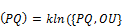(1)

where k is a scale constant, O and U chosen fixed points and {PQ, OU} is the cross ratio. Under appropriate conditions Euclidean length can be obtained from relation (1). This expression has two interesting aspects. Firstly, since length cannot be defined in reference to a standard, introduction of its dimensionality can be done by identifying k with a universal constant. The Plank length offers as a possibility. Embedding the distance expression in a coordinate framework enables definition of the fixed points. Differentiation w.r.t time gives two rates of change: rate of change of expansion, i.e. rate of change of (PQ), and rate of change of position. These are the same in Euclidean geometry. Rate of change of position will be used as the appropriate definition for velocity. Interestingly, rate of change of expansion varies with position.

Classical physical theories have a simple global structure: their mathematical formulation is founded on a geometry which describes the structure of space and time. The same schemata will be maintained but with the geometrical alterations being proposed. Connection with the mathematical apparatus of quantum mechanics is most directly made via Dirac’s q-number formulation. The algebra of space and time points form a division ring as does Dirac q-numbers. Their isomorphism is the critical geometrical-algebraic connection. The mathematical apparatus of QM can then be assumed to be built on the dual geometry being proposed in a manner not dissimilar from classical theories and their foundational metrical geometries.

Non-commutative algebra is the "cornerstone" of the mathematical apparatus of QM. The physical basis of non-commutation is found here to be a direct consequence of the structure of space and time, and the describing geometry.

A "neo classical" quantum mechanics implies that it is meaningful to associate position directly with a point in physical space. A quantum system will then be specified by the projective wave function Ψ(q), where the symbol q denotes position. Several issues immediately arise. Firstly, that of algebraic representation: there is no single representation. Appropriate representation needs to be chosen in the context of all variables involved and the commutation relations between them. According to the first axiom of QM the wave function defines all measurable quantities of the quantum system. Adhering to this postulate raises the question of measurability of the projective wave function.

For purpose of comparison it is worthwhile recapping standard QM: x denotes the eigenvalue of an operator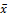which represents the position observable in Hilbert space. Only after measurement does x denote the physical particle position. Algebraic representation of the position operator is also chosen in context with other observables. However, for q-points commutation relations are a geometrical consequence. This difference is of primal importance. Mathematically q-points have the same algebraic representation as position operators in standard QM, yet physically, have the "realism" property of Newtonian mechanics; i.e. represent a real point in real physical space. Since q will then represent a counterfactual definite quantity the "measurement apparatus" construct of orthodox QM is unnecessary. Nevertheless, the measurability of q needs appropriate geometrical definition.

Clearly, the wave function in the Schrödinger (and Dirac) equation is a function of x (in position representation) in Euclidean space. To meet the requirements of the first axiom the wave function must be defined in a metrical geometry. So the obvious question arises that if space is ultimately projective how does it become Euclidean; specifically, how does Ψ(q) become Ψ(x)?

Firstly, it should be clarified that a fundamental aspect of the wave function has been implicitly assumed. Namely, the wave function has a one-to-one correspondence with an "element of reality"; in this case position. Currently, there is discussion on the nature of the wave function as either a mathematical construct or a representation of "reality" [21,22]. Conceptually, in agreement with findings this proposal concurs with the latter.

Concerning the relation between geometries, technically, if ideal points are omitted, then Projective geometry reduces as special case to Euclidean. However, the Kleinian view of geometry is more appropriate in this instance. Accordingly, geometry is viewed as a set of points and a group of allowable transformations, where properties invariant under transformations define the properties of the geometry. The most general projective transformation, where only the cross ratio is invariant, is given by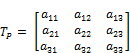(2)

A set of points subject to such a transformation will be non-Euclidean: distance, length, areas are not preserved. An important special case is the isometric transformation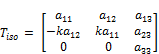(3)

Defining the matrix elements: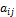are real, k2 = 1 and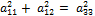This transformation defines translation and rotation. A subset of points subject to the isometric transformation will possess Euclidean invariant properties; in which sense the subspace will be "Euclidean" .

Isometric symmetries of QM are based on several fundamental physical properties. Firstly, homogeneity and isotropy are properties of space and time, and hence the describing geometry. Both are maintained in Projective geometry. Secondly, the transformed wave function must represent the same physical state – eigenvalues, expectation values and all measured quantities predicted by QM must not change. Further, definitions are introduced where S denotes the physical system represented by functions and operators. The symmetry transformation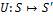acts on points while the corresponding symmetry operator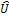acts on the wave function. From these physical principles and definitions the mathematical properties of symmetry invariance follow.

Mathematically, the same results are obtained for Ψ(q) in place of the usual Ψ(x). This has a most significant consequence. The symmetry isometric transformation acting on a quantum system is just the isometric projective transformation (3). Hence the describing geometry of the quantum system is Euclidean invariant in its properties, in which sense it can be characterized as "Euclidean". The wave function, and its associated quantities, is then embedded in a "Euclidean" subspace. Measurability of the quantum system is hence reproduced.

Derivation of the isometric symmetry conditions for the standard wave function Ψ(x) assumes a pre-measurement association (although mathematically unspecified) of x with a point in Euclidean space. Without this implicit assumption there are added complications for the "measurement apparatus" construct of orthodox QM. Not only must the "apparatus" actualize the physical position, but also the embedding Euclidean space structure. A passive, i.e. Newtonian, association with the geometry of space would circumvent added complexity.

These "measurement" complications do not arise with this proposal. However, isometric symmetry is influential in defining characteristics of the embedding space and its describing geometry. This implies an active, i.e. non-Newtonian, association between symmetry, space structure and geometry. Since non-commutation is found to arise from the structure of space and time this is not an unexpected result.

No changes are being proposed for the structure of time. As a general property, the asymmetry of space and time in standard QM - time is not represented by an operator - is now explained as arising from a different structure requiring different algebraic representation.

3. Pilot Wave Hidden Variables Model

Bohmian mechanics is a hidden variables theory first introduced by de Broglie based on a pilot-wave model of quantum "motion". Bohm subsequently re-formulated the concept [23,24]. Bell, who had reservations about interpretational aspects of QM, especially the measurement problem, found the theory interesting. But, it was the foundations of the proposed mechanics not its mathematical formulations which captured his attention. Firstly, the theory should not have existed. Hidden variables were supposed to not be possible according to the impossibility proofs on the completeness of QM. These were subsequently shown to be flawed. Further, the pilot-wave model endowed the quantum particle with an inherent deterministic trajectory. This aspect resolved the "measurement question" by the "problem" not arising. There is nevertheless, departure from classical mechanics: the theory is non-local and the variables contextual. Non-locality was the feature which most intrigued Bell, becoming the motivation for his much though-over inequalities.

The objective of the following simple pilot-wave model is also to explore foundational questions rather than theory-building.

Mathematically, Bohmian mechanics is defined by two evolution equations: the usual Schrödinger equation, together with what is termed the guiding equation. The latter describes the motion of the generic kth particle in an ensemble of N particles. While the proposed geometrical alterations do not affect the Schrödinger equation they do effect the guiding equation. Since this equation describes the time evolution of the particle space position, it is founded on a dual geometry. It will be assumed that the motion is essentially Newtonian. Accordingly, the particle velocity, taking the rate of change of position as definition, is given by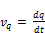(4)

Before proceeding, it is constructive to examine aspects of the orthodox interpretation of quantum mechanics which are directly pertinent to the physical veracity of the "Newtonian" relation just introduced. According to the positivist Bohr-Heisenberg construct the physical basis of this kinematics is contrary to the uncertainty principle, and therefore empirically meaningless!

Irrespective of the issues over weak measurement experiments of the intuitive Heisenberg relation, the precision uncertainty formulation due to Kennard has been experimentally verified [10,11]. As is well known, the Kennard relation refers to an ensemble of measurements with "uncertainty" being standard deviations from mean values. Consequently, this quantum mechanical formulation of the uncertainty principle can be interpreted to prohibit an individual particle path, as par Bohr-Heisenberg. Or, as with Bohm-de Broglie, the same relation can be interpreted to refer to statistical ensembles, and make no statement about individual paths. The choice becomes a matter of philosophical orientation.

With the Heisenberg relation, referred to as "error-disturbance" or "measurement-disturbance", interpretation is somewhat more complex. Heisenberg’s celebrated thought experiment was motivated to demonstrate that a path trajectory is operationally non-measurable, and therefore a concept without place in a physical theory. This fundamental principle can be invoked with whatever error-disturbance relation is experimentally verified. As will be discussed however, this principle will not be upheld in this work.

Non-simultaneous measurability of position and momentum is a different question and, within the context of this proposal, is explained geometrically. To the extent that the classical "state" is characterized by a point in phase space, simultaneous measurement of position and momentum is possible since both variables are underpinned by a common Euclidean space. Correspondingly, the quantum state is characterized by the wave function which is a function of either position or momentum but not both. As has been shown, the wave function in position representation is embedded in a Euclidean subspace, in which case position measurability is definable but not simultaneously with momentum. While the geometry of the wave function in momentum space has not been considered it is expected to mirror that of position. Non-simultaneous measurability is more directly manifest in the position-momentum commutation relation, which prohibits simultaneously representing both variables as metric-type.

Newtonian assumptions will continue to be made in the following treatment. For periodic motion of period τ, the probability density for position is given by(5)

This refers to a particle with speed v(q), spending a time dt, in a small region of space dq, near a point q (a description invalid in orthodox QM). For a simple one dimension model (may be more general)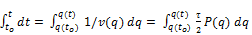(6)

While the probability density function is unknown, by definition P(q) gives the probability of the particle being at position q. However, this must also be the probability of the particle being at point x; as they are the same physical point. Hence P(q) must have a direct relation with │Ψ(x)│2 which also ensures that the probability in q-space is a real number. Accordingly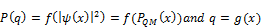(7)

A further extension is necessary, introducing an entangled particle pair, giving,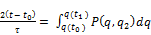(8)

where q2 refers to the position of the entangled other particle. Obviously, there is also a mirror relation for the entangled partner giving a system of coupled equations. While the functional relations of (7) are not known, for the purposes of this analysis it suffices that such functions exist. Integrating gives,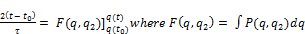(9)

After appropriate substitutions the relation becomes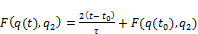(10)

While the mathematical form of this relation is complex, and is further complicated by obtaining the inverse function of F(q, q2 ), its underlying relational structure is quite simple. Namely;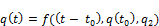(11)

Assuming the existence of the function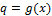and its inverse, it is possible to obtain the complementary expression for the particle trajectory in Euclidean space. For projective space, particle position at any given time is a function of a time interval, initial conditions and the position of the entangled other particle. Since the RHS of relation (11) involves the position of the entangled partner it may suggest that the non-locality feature remains. But this is not the case. For this periodic model the feature being expressed is synchronicity not non-locality.

This can be seen by considering the following: suppose the idealized situation where at time t1 the particle pair instantaneously separate such that entanglement is broken. After separation the probability density function becomes a product function such that the probability of either particle being at any given position is independent of the other. Generic functional relations for the time sequence t ≥ t1 > t0 are given by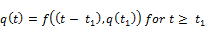(12)(13)

During the interacting period the particle is indeed dependent of the position of its partner but this is no longer the case in the non-interacting period. This implies the entanglement of the particles is not holistic but merely synchronized. However, since the initial conditions of the separated system are the final conditions of the entangled particles, the entangled state will still influence the particle position after separation. But this is solely an initial-conditions mechanism not requiring non-local influences.

Contextual representation of variables remains. This is because the algebraic representation of any variable has to been chosen in conjunction with all other variables and the commutation relations between them. Interestingly, if it can be reasoned that time has a non-metric structure then it too becomes open to non-metric algebraic representation. A possibility is to represent q-points by quaternions. This would then define the relation. However, the Edmonds relations apply for position in spacetime; corresponding relations will need to be determined for the geometry being proposed. Nevertheless, since spin would be incorporated with particle position the initial- conditions mechanism of relations (12) and (13) would also apply to the spin state.

4. Bell Inequalities

While there is a depth of analysis for experimental configurations where there is violation, cases where Bell inequalities are in agreement with QM have received little attention. Bell’s last work on inequalities was with free particle position-momentum variables, a configuration most like the original EPR thought experiment .

Space and time variables inequalities also exist for position-position measurements at different times, and position-momentum measurement for particles in a potential; violation is found with both configurations. But no violation is found for position-momentum inequalities for free particles [25,26,27,28]. This pattern of violation can be explained by the assumption of metric variable-type: where this assumption is valid, namely free particles, there is no violation, where the assumption is not valid, namely bound and time different measurements, there is violation.

Most experimental and theoretical attention is devoted to spin states. Proof of the Abner Shimony CHSH-Bell inequality will be considered . Conditional probabilities are defined as follows: p1 (s│a,b,t) refers to the probability that the outcome of the measurement performed on particle 1 is s, measurements performed on 1 and 2 respectively are a and b, with the result of the experiment on 2 is t. For particle 2 the corresponding probability is then p2 (t│a,b,s), while p(s,t│a,b) is the probability that the results of the joint measurements a and b are s and t respectively. Two independence assumptions are introduced. Parameter independence gives p1 (s│a,b,t) = p1 (s│a,t) i.e. outcome for 1 is independent of b, with a corresponding probability relation for particle 2. Outcome independence gives p1 (s│a,t) = p1 (s│a) i.e. outcome for 1 is independent of t, with a corresponding condition for particle 2. Locality is understood as a conjunction of the two independence conditions. Combined, these conditions are equivalent to the factorization condition p(s,t│a,b) = p1 (s│a,) p2 (t│b). By simultaneously assigning number values to all outcomes although not actually simultaneously measured, i.e. the assumption of metric variable-type, expectation values are calculated and the inequality follows.

Proof of the same inequality is also available which follow Bell by introducing a hidden variables parameter; which some suggest is superfluous. This parameter, usually denoted by λ, is said to represent the "microstates", or "hidden variables" or "additional data set" which completely specifies the system. Other terminology is also used, while Bell referred to "causes". Conceptually, the idea has its origins in the EPR paradox.

A most general formulation of this type introduces two control parameters,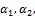and two real-valued measurement outcomes A1, A2 . Control parameters randomly determine which of possible measurements are actually performed. A general probability distribution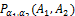defines the system. Again, a factorization condition is introduced: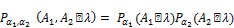(14)

Two assumptions are identified: parameter independence and local causality. Parameter independence assumes that settings at one location do not influence settings and outcomes at the other locations. Locality causality is somewhat more subtle. Firstly, it is said to not imply the independence condition: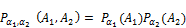(15)

Indeed, "it is perfectly natural to expect that previous interaction between the systems 1 and 2 could produce dependence". Rather, "locality requires that some data set λ – made available to both systems, say, by a common source – must fully account for the dependence between A1 and A2 " . Introducing the product A1 * A2, with outcomes simultaneously taking possible values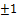, expectation value are calculated and the CHSH inequality follows. Again the assumption of metric variable-type is implicitly made.

Concerning the inoperative independence condition (15), it seems to contradict Outcome independence of the Shimony treatment. More significantly, however, the reason given for its invalidity, that of previous interaction, concurs with the initial-conditions mechanism of BM.

Before exploring the role of the hidden variables parameter it is important to be very clear on the nature of Bell’s theory. The theory is not data analysis; where experimental outcomes are direct input to statistical techniques used to extract underlying relations between variables. Nor is the theory an operational analysis of experiment leading to a conjuring of counterfactual paradoxes. Rather, the theory is a mathematical modeling of experiment, in which sense it is a "physical theory", subject to issues of variable representation. Experiment outcome are assumed to be metric variable-type i.e. taking number-values, thereby forming a field, and having, as a mathematical property, measurability, irrespective of whether they are actually measured.

On first impression it may appear that outcomes, being eigenvalues, meet both conditions. However, this is not the case. Eigenvalues are extracted from variables which in general are non-metric, most notably spin. The mathematical apparatus of QM obtains for non-metric variables both the number-value of their eigenvalues, as well as define the appropriate conditions for measurability. Dirac refers to obtaining c-values from q-values. It is not simply a mathematical procedure of converting a non-metric variable, representing a physical quantity, to metric, yet somehow representing the same quantity.

Questions on the universality of the hidden variables parameter, and attributing factorization solely to locality, are peripheral to this discussion. The assumption of metric variable-type is made in calculating expectation values. Further, it will be accepted that inclusion of the parameter in conditional probability definitions enables defining constraints on the nature of possible hidden variables theories.

Violation can then also be interpreted to disallow hidden variables theories which algebraically represent physical quantities by metric variable-type. The "hidden variables theory" being proposed here is not this type of theory.

A "dissent" analysis is worth considering . Mathematically, the analysis follows Bell’s EPR free particles work utilizing the non-negativity of Wigner functions . Concerning the all-important underlying assumptions, the authors argue that while "locality is not an issue", two explicit assumptions are made: "(1) independence of A on the settings of B (and vice versa) and (2) each dynamical variable has values for the observables, whether or not they are simultaneous measured."

Firstly, the work re-affirms Bell’s expectation that correlations of observables for the EPR state "would not allow for BIQV" i.e. inequality violation. More generally, especially in reference to CHSH, the authors assert that "it is really the second assumption which leads to Bell’s inequality – whose violation, therefore, implies the theory disallows it."

The main conclusion is that Bell’s inequality "hinges on the assumption of having definite values for all the dynamical variables – thus endowing them with physical reality – and not the issue of locality." Implicit in the first premise is again the assumption of metric variable-type. The inference of the second premise, that "physical reality" is endowed to a dynamical variable by associating to it a definite number-value, is false. Physical characteristics of a particle i.e. position, momentum, spin, can be algebraically represented by non-metric variables without denying their inherent reality.

5. Quantum Lorentz Transformations

Special Relativity is based on a fundamental postulate referred to as the Principle of Relativity, which imposes departure from basic physical characteristics of Newtonian mechanics. Geometrically, however, space and time remain described by metrical i.e. Euclidean geometry. Further, relativity remains "Newtonian" in the sense that it maintains the reality of a particle path and description of phenomena is space and time. Spatial and temporal dimensions are merged into the Minkowski notion of spacetime. Recently, however, this concept has come under question . Quantum mechanics, of course, differs radically from classical theory; at least according to its orthodox interpretation. Although reasons are available to suggest otherwise, the "tension" with relativity is deepened with the commonly accepted reason for the violation of Bell inequalities . Non-compatibility of the two grand theories makes finding conceptual consistency difficult. Consistency, however, is desirable if it is reasonably assumed that nature is defined from a common set of underlying physical principles.

Within the context of this proposal there is foundational common ground between the theories. Quantum theory is essentially "Newtonian" in maintaining realism, determinism and locality; as well as a physically real space and time motion. Departure from classical theory is in the details of the geometry. As will be discussed, measurability, however, does present a fundamental difference from classical thinking. A neo-classical quantum mechanics has the simple interesting consequence that "quantum" Lorentz transformations become physically meaningful. In the micro-domain being proposed, Lorentz transformations would be subject to the "relativity principle", a requirement of special relativity, and also be subject to division ring algebra for position and velocity variables, as required by quantum mechanics.

Mathematically, of course, there is no impediment to obtaining modified transformations subject to a different algebra. The issue is one of physical meaning. Within the framework of current orthodoxy it is difficult to envisage what physical reality could be attributed to such mathematical constructions.

Standard derivations of the LT are founded on a further postulate, namely, constancy of the speed of light. However, the procedure utilized here follows those derivations based on the homogeneity and isotropy of space and time together with the relativity principle [31,32,33]. Group properties of the LT are also used as a mathematical condition. These derivations give rise to a free parameter, with dimensionality of speed. Identification with the speed of light, and implications for the veracity of spacetime concept, will not be pursued here [31,32].

The usual relativistic assumptions apply in the following: two inertial reference frames O and O`, moving at relative speed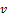along the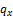axis, with coordinate transformations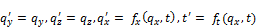(16)

Since the space-geometry is now projective, position coordinates obey a non-commutative algebra w.r.t the relative speed. Homogeneity of space and time require the transformation functions to be linear. Thus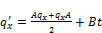(17)(18)

As usual, the coefficients A, B, C, D are functions of the relative speed but with the modification of non-commutation included in the expressions. These coefficients are then obtained by considering several special-case conditions. Firstly, the origin of O` has coordinate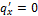and moves with velocity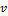relative to O, so that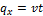; where the relative velocity is defined as the rate of change of position. Applying some basic algebra gives the first relation between coefficients as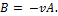Alternatively, the origin of O has coordinate q = 0 and moves with a negative relative velocity relative to O`. Applying this condition results in D = A. Introducing the more usual notation A = γ, and the new variable E = CA-1the relations become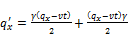(19)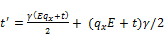(20)

The next special case condition is to consider two subsequent transformations where, because of the group property of LT, the combined transformation is also a Lorentz transformation. Since, for a general LT the coefficients of q and t are equal, the condition follows that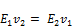(21)

In which case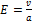where a is a constant to be identified. The transformations now become(22)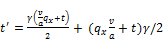(23)

The next special-case condition, to determine the γ function, is to make a transformation followed by the inverse. For the time coordinate this leads to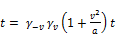(24)

So that(25)

Because of space symmetry the two gamma functions must be equal, and identifying the constant,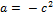, gives the usual form. An interesting complication emerges in the final special-case condition for the position coordinate giving the relation(26)

Clearly, the coefficients on the RHS appear problematic. However, introducing the Feynman bracket (although non-relativistic)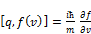(27)

Re-arranging the ordering of the position and velocity terms gives the required result of unity for the RHS coefficient. The final transformations are(28)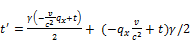(29)

Under appropriate geometrical conditions the standard classical equations are obtained.

Two signification extensions follow: the addition of velocities and "relativistic" mass relations. Following standard algebraic procedures, while assuming all velocities variables commute, gives relations which are unchanged in their mathematical form. The critical difference, however, is that momentum and velocity variables are no longer metric-type.

Invariance of the space-time and energy-momentum 4-vectors is somewhat more complicated. Since the latter relation involves only velocity dependent terms, all of which commute, the energy-momentum 4-vector remains invariant. This is expected since the rest mass is the same in all reference frames. The space-time 4-vector is, however, found to not be invariant. Mathematically this is not an unexpected result. Invariance of the space-time vector in standard relativity is a consequence of the Minkowski spacetime metric; which does not apply to the Projective-Euclidean dual geometry for space and time being proposed.

As a general condition space and time in the proposed new structure are treated differently. This raises potential contradiction with experimentally verified fundamental configurations where space and time are treated equivalently. As has been shown, the wave function in position representation is embedded in a Euclidean subspace. Position (as defined in the wave function) is then Euclidean, as is time. Hence in this special case configuration the treatment of space and time are geometrically equivalent as Euclidean. The asymmetry of space and time manifests in the path description, i.e. position as a function of time, but this aspect is not described by the wave function for which it is a "hidden variable".

Space and time equivalence deepens with relativity. Again, there is no contradiction for quantum phenomena described by the wave function (which at present is all quantum phenomena). For the wave function the relative inertial frames are "Euclidean". Relativistic invariance conditions for the Dirac equation remain unchanged.

While the implications of this proposal for Quantum Field Theory would require deeper consideration, it cannot be assumed that space and time asymmetry will cause contradiction. Again, it becomes a question of defining a special case geometrical configuration which underpins QFT.

6. Measurement

The central concept of this discussion is to understand quantum mechanics within a non-metric structure of space. Conceptually, this minimizes the departure from classical mechanics to mainly alterations in the underlying geometry. While there is no longer a need to introduce a complex metaphysics, there is nevertheless a fundamental shift from classical understanding. A non-metric space introduces concepts whose measurability cannot be geometrically defined directly. Consequently, accepting such a structure requires accepting that measurement is a secondary aspect of "nature". Only under appropriate conditions is measurement definable. This presents an immediate contradiction with the principle that immeasurable concepts have no place in a physical theory.

However, this "principle" is itself a philosophical thought tool only. Indeed, in the axiomatic formulation of quantum mechanics it was disused in the first axiom. The then non-measurable wave function was nevertheless postulated to describe all that was measurable of a quantum state.

Measurability is obviously a deep question in natural philosophy and its rejection as fundamental is to be carefully considered. However, it is important to emphasize the conservatism of this re-definition. Measurability remains defined within space and time. Further, measurability is passive; observation does not create or bring into being "an element of reality". The latter contrasts with the orthodox interpretation where measurement is an intrusive procedure actualizing an element of reality, for example position, from a "super-reality" of potentialities. Departure from classical definition is restricted to Euclidean metric properties being founded on more fundamental invariant generic conditions.

There are nevertheless parallels with aspects of orthodox concepts. While the need to introduce a "measurement apparatus" is circumvented, there is no return to simple classical measurability. Measurability of the wave function is conditional on imposing a "Euclidean" overlay on a projective subspace, as defined by quantum isometric symmetry. Physical quantities which then become defined as measurable can be measured classically. Orthodox QM interprets measurement as arising from the interaction of the quantum micro-domain with the classical macro-domain. Measurability here emerges from the interface of projective space with a "classical" Euclidean subspace. An interesting possibility arises as to whether the probabilistic feature of QM results from a loss of path measurability (not a loss of realism) in transition between the two geometrical spaces.

Nevertheless, reservations are understandable. However, as has been previously stated, unless an explanation is found to show otherwise, violation of Bell inequalities requires some fundamental aspects of classical understanding to be revised. Currently the considered options are: rejection of "locality" (the sole option for some) or rejection of "realism" (unpalatable to many if not most). This proposal would appear the least radical alternative. In what may be more significant however, it enables definition of a core set of non-contradictory physical characteristics common to both quantum mechanics and relativity.

While Bell’s theory has attracted enormous attention it is nevertheless a secondary question only. The primary question remains whether quantum mechanics can be more completely described by a deeper structure. Bell initially showed that such a structure was possible, contrary to impossibility proofs in vogue at the time. The motivation for asking such a question was (and still is) a legitimate disquiet by some on the radical departure from "common sense" notions of reality that quantum mechanics seemingly implies. Bell subsequently showed that even with a more complete description some "unsavory" features persisted. This proposal offers two potentialities: a more conservative (locality and realism are preserved) interpretation on the violation of Bell inequalities, and a possible "neo-classical hidden variables" structure. However, all options are open to consideration.

7. Conclusion

A key foundational concept in the early development of quantum mechanics was that then then "new mechanics", was to be formulated without reference to a particle path. Since orthodox quantum mechanics has been found to be a matter of philosophical choice rather than necessity the question arises whether such a radical departure from classical mechanics is required. If the idea of a particle path is resumed then some kind of space and time description would follow. This proposal offers a possibility.

New ideas are seldom correct. And, it would be foolish to not be mindful of this balance of probabilities. However, exploring new hypothesis remains a necessity; even for physical theories of such empirical grandeur as quantum mechanics. Especially, perhaps with quantum mechanics, where after almost a century there is still unease as to what it physically means.

References

1. J. S. Bell, Speaking and Unspeakable in Quantum Mechanics (Cambridge University Press, Cambridge, UK 1987)
2. A. Aspect, P. Granger and G. Roger, Phys. Rev. Lett. 49 n. 2, p. 91 (1982)
3. T. M. Nieuwenhuizen, Found. Phys. (2011) 41: 580-591
4. Travis Norsen, Found. Phys. (2007) 37: 311-339; T. Norsen, American Journal of Physics 79, 1261 (2011)
5. H. M. Wiseman, J. Phys. A. Math. Theor. 47 (2014) 424001at http://arXiv.org/abs/1402.0351 (2014)Howard M. Wiseman and Eric G. Cavalcanti, at http://arXiv.org/abs/1503.06413v1 (2015)Travis Norsen International Journal of Quantum Foundations 1: 65 – 84, 2015
6. Marek Zukowski, at http://arXiv.org/abs/1501.05640v1 (2015), http://arXiv.org/abs/1501.04618v1 (2015)
7. A. Aspect, Nature 438, 745 (2005)Nicolas Gisen, Found. Phys. Vol. 42, no. 1, 80-85 (2010)
8. G. Ghirardi, Journal of Physics: Conf. Ser. 174 (2009) 012013
9. S. Goldstein, T. Norsen, D. Tausk, N. Zanghi, Scholarpedia, 6 (10): 8378
10. M. Ozawa, Phys. Lett. A. 318 (2003) 21; Masanao Ozawa, at http://arXiv.org/abs/1308.3540v1 (2013)
11. J. Erhart, S. Sponar, G. Sulyok, G. Badurek, M. Ozawa, and Y. Hasegaw, Nature Physics vol 8, 185 (2012)
12. Paul Busch, Pekka Lahti, Reinhard F. Werner, at http://arXiv.org/abs/1312.4392v2 (2014)
13. H. M. Wiseman, 2007 New J. Phys. 9, 165 doi:10.1088/1367-2630/9/6/165
14. S. Kocsis, B. Braverman, S. Ravets, M. J. Stevens, R. P. Mirin, L. Krister Shalm, A. M. Steinberg, Science, vol 332 1170 (2011)
15. J. S. Lundeen, A. M. Steinberg, Phys. Rev. Lett. 102, 020404 (2009)
16. Fosco Ruzzene, American Journal of Modern Physics, Vol. 2, No. 6, 2013 pp. 350-356
17. David Hilbert, The Foundations of Geometry 2005 eBook 17384
18. Gilbert De B. Robibson, The Foundations of Geometry, fourth Edition, University of Toronto Press
19. C. R. Wylie, Introduction to Projective Geometry, Dover Publications, Inc. New York
20. C. R. Wylie, Foundations of Geometry, Dover Publications, Inc. New York
21. Matthew F. Pusey, Jonathan Barrett and Terry Rudolph, Nature Physics, 8, 475 – 478, (2012), doi:10.1038/nphys2309
22. Roger Colbeck and Renato Renner, at http://arXiv.org/abs/1111.6597v2, http://arXiv.org/abs/1312.7353v1
23. D. Bohm, Physical Review, 85, 166-193 (1952)
24. Bohmian Mechanics, Stanford Encyclopedia of Philosophy
25. J. S. Bell, Ann. (N. Y.) Acad. Sci. 480, 263 (1986)
26. A. M. Cetto, L. La Pena and E. Santos, Phys. Lett. 113A, 304 (1985)
27. O. Cohan, Phys. Rev. A. vol. 56. No. 5, 3484 (1997)
28. J. Kiukas, R. F. Werner, J. of Math. Phys. vol 51, no. 7, 072105 (2010)
29. Abner Shimony, Bell’s Theorem, Stanford Encyclopedia of Philosophy
30. M. Revzen, P. A. Mello, A. Mann, L. M. Johansen, Phys. Rev. A. 71 022103 (2005)
31. Luigi Maxmilian Caligiuri, Amrit Sorli, American Journal of Modern Physics. Vol. 2, No. 6, 2013, pp. 375-382
32. Palash B. Pal, Eur. J. Phys. 24, 315-319 (2003)
33. Alon Drory, at http://arXiv.org/abs/1412.4018v1
34. James D. Edmonds, Jr. International Journal of Theoretical Physics, vol. 6, no. 3, 205 (1972).

 Contents 1. 2. 3. 4. 5. 6. 7.
Article ToolsAbstractPDF(255K)